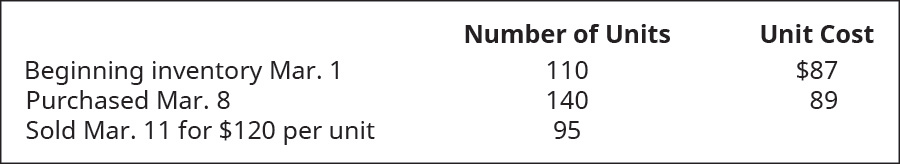# Calculate the cost of goods sold dollar value for A74 Company for the sale on March 11, considering the following transactions under three different cost allocation methods and using perpetual inventory updating. Provide calculations for (a) first-in, first-out (FIFO); (b) last-in, first-out (LIFO); and (c) weighted average (AVG).FindFindarrow_forward

### Principles of Accounting Volume 1

19th Edition
OpenStax
Publisher: OpenStax College
ISBN: 9781947172685

#### Solutions

Chapter
SectionFindFindarrow_forward

### Principles of Accounting Volume 1

19th Edition
OpenStax
Publisher: OpenStax College
ISBN: 9781947172685
Chapter 10, Problem 4PA
Textbook Problem
3 views

## Calculate the cost of goods sold dollar value for A74 Company for the sale on March 11, considering the following transactions under three different cost allocation methods and using perpetual inventory updating. Provide calculations for (a) first-in, first-out (FIFO); (b) last-in, first-out (LIFO); and (c) weighted average (AVG).To determine

(a)

Concept introduction:

Cost of goods sold: It is the cost which is also known as cost of goods manufactured, calculates the total value of goods produced during the period and is ready for sale. In other word total amount of expense incurred to turn raw material inventory into finished goods.

To calculate:

Cost of goods sold as per FIFO.

### Explanation of Solution

To calculate the cost of goods sold company multiply the cost per unit to the quantity sold...

To determine

(b)

Concept introduction:

Cost of goods sold: It is the cost which is also known as cost of goods manufactured, calculates the total value of goods produced during the period and is ready for sale. In other word total amount of expense incurred to turn raw material inventory into finished goods.

To calculate:

Cost of goods sold as per LIFO.

To determine

(c)

Concept introduction:

Cost of goods sold: It is the cost which is also known as cost of goods manufactured, calculates the total value of goods produced during the period and is ready for sale. In other word total amount of expense incurred to turn raw material inventory into finished goods.

To calculate:

Cost of goods sold as per weighted average.

### Still sussing out bartleby?

Check out a sample textbook solution.

See a sample solution

#### The Solution to Your Study Problems

Bartleby provides explanations to thousands of textbook problems written by our experts, many with advanced degrees!

Get Started